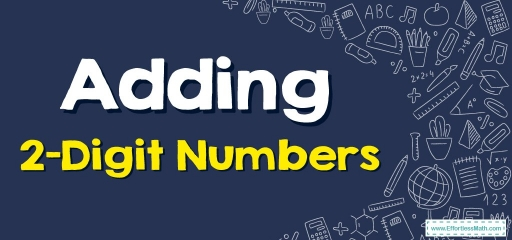Do not know the basics of adding two-digit numbers? So the following article is what you need!$$2$$-digit adding is a type of adding containing only $$2$$ digits positioned in their corresponding place values. Two-digit adding is an example of one of the simplest types of adding. Let’s find out the basics for adding two-digit numbers altogether.

## Related Topics

Two-digit adding is accomplished by remembering the place value of the numbers. Numbers having two digits have place values as ones and tens.

Whilst adding two digits altogether, you place these numbers in a column-type manner within the appropriate place value. That technique assists in adding together the digits one at a time.

Two-digit adding can be accomplished in two ways, the first is via regrouping (carrying forward) and the other is done with no regrouping (don’t carry it forward). Numbers being added are known as addends and your answer obtained after you add them together is known as the sum.

$$2$$-digit addition with regrouping is a method used when the sum of the numbers in one column is more than $$9$$, we carry forward the number to the next column.

In the case of $$2$$-digit numbers, regrouping or carrying forward occurs from the one’s column to the tens column. The basic steps to follow while adding $$2$$ digits with regrouping are:

Step one: Place the digits or addends based on their place value such as ones and tens placed column-wise or in a vertical fashion.

Step two: Begin adding your numbers starting from the right, such as the one’s column, then go towards the left, such as the tens column.

Step three: If the one’s column sum is higher than $$9$$, you get a double-digit number once more. From this double-digit number, you put the number in the one’s column underneath the one’s column, the tens number is carried forward or rearranged in the tens column of the initial numbers.

Step four: Whilst adding the numbers in the tens column, you also add the tens number which you carried forward from your one’s column.

Step five: Whenever these columns get added up, you achieve the final answer.

## 2 Digit Adding With no Regrouping

Two-digit adding with no regrouping is the easiest kind of adding. Here, whenever the sum is lower than or the same as $$9$$, you put the number underneath the respective place value column but don’t carry forward any digit to the next column. Two-digit adding with no regrouping utilizes like steps as does regrouping, yet several steps get left out. Let’s take a look at the basic steps utilized whilst doing an addition of two-digit numbers with no regrouping:

Step one: Organize the numbers or addends based on the place value, for instance, ones and tens go in column-wise or are placed in a vertical style.

Step two: Begin using addition by adding these numbers from right to left, for instance, one to tens.

Step three: When you add up both of these columns, you get the final answer.

### Adding 2-Digit Numbers – Example 1:

Find the sum.

$$\begin{array}{r} &52\\ +\!\!\!\!\!&21\\ \hline \end{array}$$

Solution:

First line up the numbers: $$\begin{array}{r} &52\\ +\!\!\!\!\!&21\\ \hline \end{array}→$$ Add the numerals in the ones’ spot column $$(2 + 1 = 3)$$ and put the resulting answer under the line. $$\begin{array}{r} &52\\ +\!\!\!\!\!\!&21 \\ \hline \end{array} \\ \ \ \ \ \ \ \ \ 3$$ , Continue with tens’ place. $$5 \ + \ 2=7$$, $$\begin{array}{r} &52\\ +\!\!\!\!\!\!&21 \\ \hline \end{array} \\ \ \ \ \ \ \ 7$$. $$\begin{array}{r} &52\\ +\!\!\!\!\!\!&21 \\ \hline \end{array} \\ \ \ \ \ \ \ 73$$

### Adding 2-Digit Numbers – Example 2:

Find the sum.

$$\begin{array}{r} &13\\ +\!\!\!\!\!&78\\ \hline \end{array}$$

Solution:

First line up the numbers: $$\begin{array}{r} &13\\ +\!\!\!\!\!&78\\ \hline \end{array}→$$ Add the numerals in the ones’ spot column $$(3 + 8 = 11)$$ you get a double-digit number once more. From this double-digit number, you put the number in the one’s column underneath the one’s column, the tens number is carried forward or rearranged in the tens column of the initial numbers. $$\begin{array}{r} &13\\ +\!\!\!\!\!\!&78 \\ \hline \end{array} \\ \ \ \ \ \ \ \ \ 1$$ , Continue with tens’ place. Whilst adding the numbers in the tens column, you also add the tens number which you carried forward from your one’s column, $$1 + 7+1=9$$, $$\begin{array}{r} &13\\ +\!\!\!\!\!\!&78 \\ \hline \end{array} \\ \ \ \ \ \ \ 9$$. $$\begin{array}{r} &13\\ +\!\!\!\!\!\!&78 \\ \hline \end{array} \\ \ \ \ \ \ \ 91$$

## Exercises for Adding 2-Digit Numbers

Find each sum.

1. $$\color{blue}{63+25}$$
2. $$\color{blue}{44+54}$$
3. $$\color{blue}{38+24}$$
4. $$\color{blue}{85+12}$$
1. $$\color{blue}{88}$$
2. $$\color{blue}{98}$$
3. $$\color{blue}{62}$$
4. $$\color{blue}{97}$$

### What people say about "Adding 2-Digit Numbers - Effortless Math: We Help Students Learn to LOVE Mathematics"?

No one replied yet.

X
30% OFF

Limited time only!

Save Over 30%

SAVE $5 It was$16.99 now it is \$11.99